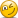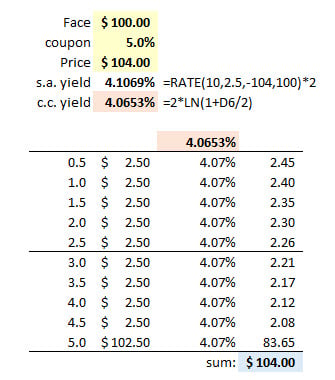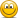# Function for computing continuously compounded yield on BA II Plus Pro

#### Jagan.Ganti

##### New Member
Hi

In reference to the following question from Hull - I'm wondering if there is a way of directly computing continuously compounded yield (CCY) using a TI BA II Plus Pro.

"4.27 A five-year bond provides a coupon of 5% per annum payable semi-annually. Its price is 104. What is the bond's yield? You may find Excel's Solver useful."

Just checking if my approach to solving this using a BA II Plus (since excel is not permitted on FRM) is correct.

Step1: PV=-104, Fv=100, N=10, PMT=5/2, => solving for Y=2.053% (semi-annual, m=2);
Step2: CCY = m*ln(1+Y) = 2* ln(1+2.053) = 4.0653%;

If not, request to be corrected.

Thank you.
Jagan

#### David Harper CFA FRM

##### David Harper CFA FRM
Staff member
Subscriber
Hi @jag.aus.gvs Yes, that works because it must workand i think it's the only way to solve it with the calculator. If there is any doubt, see my XLS (screenshot below) https://www.dropbox.com/s/1a78rvjv697wgjq/011221-hull-4-27.xlsx?dl=0 Thanks,#### Jagan.Ganti

##### New Member
Many thanks, David